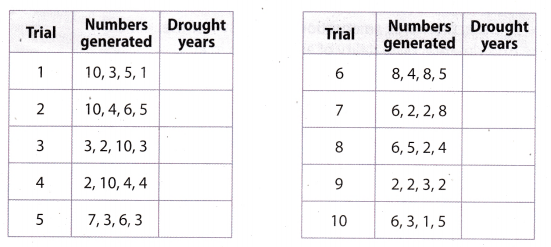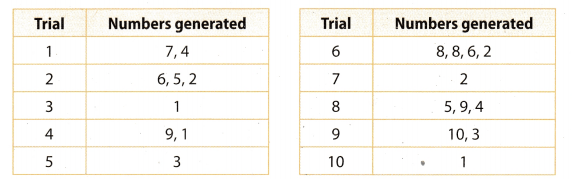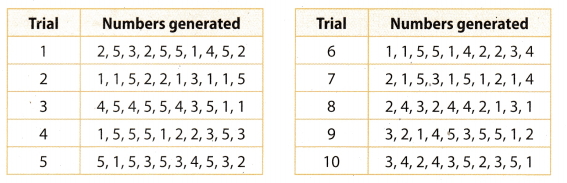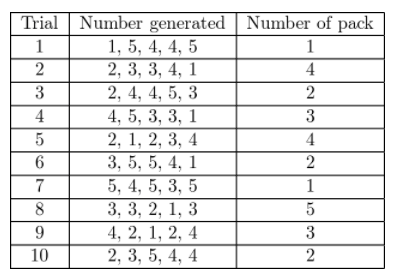# Texas Go Math Grade 7 Lesson 6.4 Answer Key Using Technology to Conduct a Simulation

Refer to our Texas Go Math Grade 7 Answer Key Pdf to score good marks in the exams. Test yourself by practicing the problems from Texas Go Math Grade 7 Lesson 6.4 Answer Key Using Technology to Conduct a Simulation.

## Texas Go Math Grade 7 Lesson 6.4 Answer Key Using Technology to Conduct a Simulation

Question 1.
An elephant has a 50% chance of giving birth to either a male or to a female calf. Use a simulation to find the experimental probability that the elephant gives birth to 3 male calves before having a female calf. (Hint: Use 0s and 1s. Let 0 represent a male calf, and 1 represent a female calf. Generate random numbers until you get a 1.)The probability that the elephant gives birth to three male calves before having a female calf is $$\frac{7}{10}$$.

Texas Go Math Grade 7 Solutions Lesson 6.4 Answer Key Question 2.
Matt guesses the answers on a quiz with 5 true-false questions. The probability of guessing a correct answer on each question is 50%. Use a simulation to find an experimental probability that he gets at least 2 questions right. (Hint: Use 0s and is. Let 0s represent incorrect answers, and 1s represent correct answers. Perform 10 trials, generating 5 random numbers in each, and count the number of 1s.)The probability to get at least 2 question right is $$\frac{7}{10}$$.

There is a 30% chance that T’Shana’s county will have a drought during any given year. She performs a simulation to find the experimental probability of a drought in at least 1 of the next 4 years. (Examples 1 and 2)

Question 1.
T’Shana’s model involves the whole numbers from 1 to 10. Complete the description of her model.
Let the numbers 1 to 3 represent _____________ and the numbers 4 to 10 represent _____________
Perform multiple trials, generating _______ random numbers each time.
Let the numbers 1 to 3 represent that will be drought during any given year, and the numbers 4 to 10 represent that will not have drought during any year.
Perform multiple trials, generating 4 random numbers each time.

Question 2.
Suppose T’Shana used the model described in Exercise 1 and got the results shown in the table. Complete the table.The probability that there will be a drought in the country at least 1 of the next 4 years is $$\frac{8}{10}$$.

Question 3.
According to the simulation, what is the experimental probability that there will be a drought in the county in at least 1 of the next 4 years?
The probability that there will be a drought in the country at least 1 of the next 4 years is $$\frac{8}{10}$$.

Essential Question Check-In

You want to generate random numbers to simulate an event with a 75% chance of occurring. Describe a model you could use.
The probability of some event is = $$\frac{75}{100}$$ = $$\frac{3}{4}$$.
We can use numbers 1, 2, 3 which represent that the chosen event is happen and 4 that is not.
Generating a random number in every trial until you get 1 or 2 or 3.

Every contestant on a game show has a 40% chance of winning. In the simulation below, the numbers 1-4 represent a winner, and the numbers 5-10 represent a non winner. Numbers were generated until one that represented a winner was produced.Question 5.
In how many of the trials did it take exactly 4 contestants to get a winner?
Only in one. In the eighth trial, it was taken exactly 4 contestants to get a winner.

Based on the simulation, what is the experimental probability that it will take exactly 4 contestants to get a winner?
The probability of taking exactly 4 contestants to get a winner is $$\frac{1}{10}$$.

Over a 100-year period, the probability that a hurricane struck Rob’s city in any given year was 20%. Rob performed a simulation to find an experimental probability that a hurricane would strike the city in at least 4 of the next 10 years. In Rob’s simulation, 1 represents a year with a hurricane.Question 7.
According to Rob’s simulation, what was the experimental probability that a hurricane would strike the city in at least 4 of the next 10 years?
The experimental probability that a hurricane would strike the city in at last 4 of the next 10 years is $$\frac{2}{10}$$ = $$\frac{1}{5}$$.

Question 8.
Analyze Relationships Suppose that over the 10 years following Rob’s simulation, there was actually 1 year in which a hurricane struck. How did this compare to the results of Rob’s simulation?
The probability that over the 10 years, where was 1 year in which hurricane struck is $$\frac{3}{10}$$.
How is the experimental probability of Rob’s simulation $$\frac{1}{5}$$, the probability that over the 10 years, where was 1 year in. which hurricane struck is greater.

Question 9.
Communicate Mathematical Ideas You generate three random whole numbers from 1 to 10. Do you think that it is unlikely or even impossible that all of the numbers could be 10? Explain?
The probability that in three generated numbers all of three is 10 is:
P (in each is 10) = $$\frac{1}{1000}$$
Because we have 10 possibilities for first ∙ 10 possibilities for second ∙ 10 possibilities for third = 1000, but only one that all of three are 10.

Erika collects baseball cards, and 60% of the packs contain a player from her favorite team. Use a simulation to find an experimental probability that she has to buy exactly 2 packs before she gets a player from her favorite team.The probability that she has to buy exactly 2 packs before she gets a player from fer favorite time is $$\frac{3}{10}$$.

H.O.T. Focus on Higher Order Thinking

Question 11.
Represent Real-World Problems When Kate plays basketball, she usually makes 37.5% of her shots. Describe a simulation that you could use to find the experimental probability that she makes at least 3 of her next 10 shots.
The probability that Kate makes a shot is $$\frac{3}{8}$$.